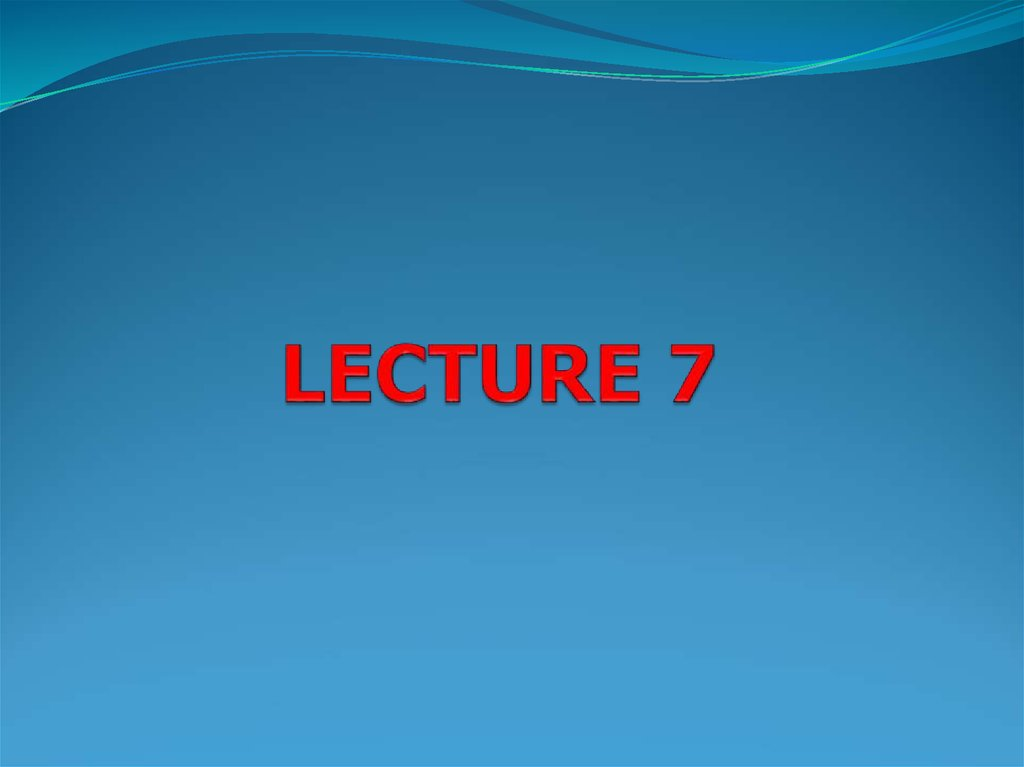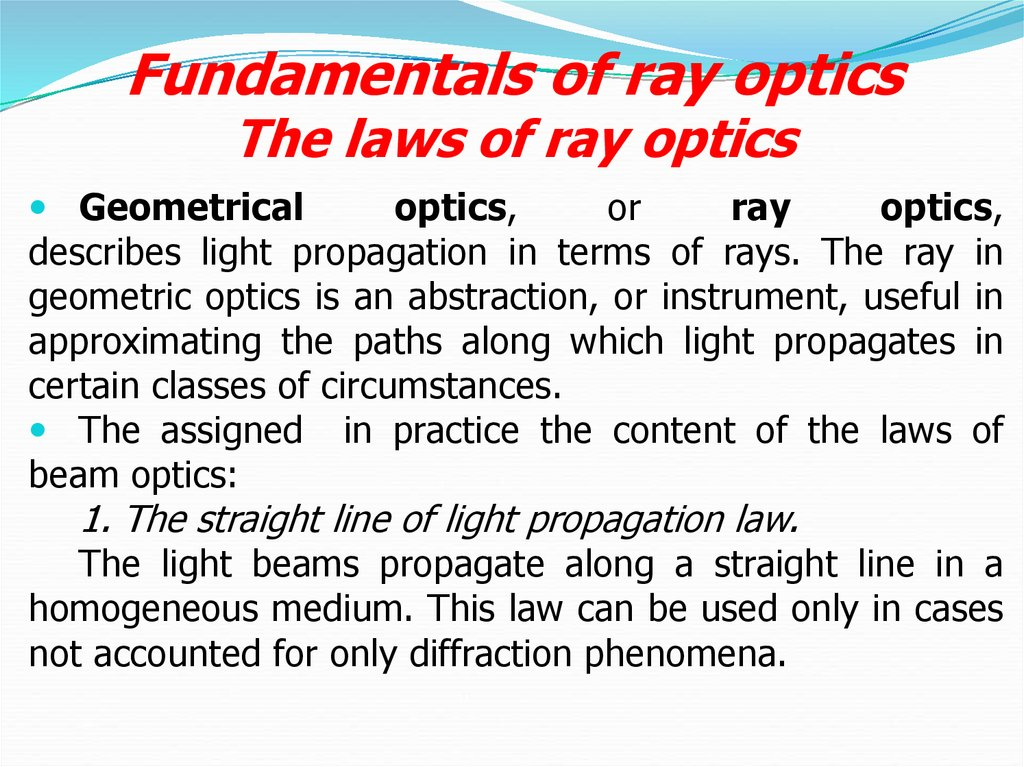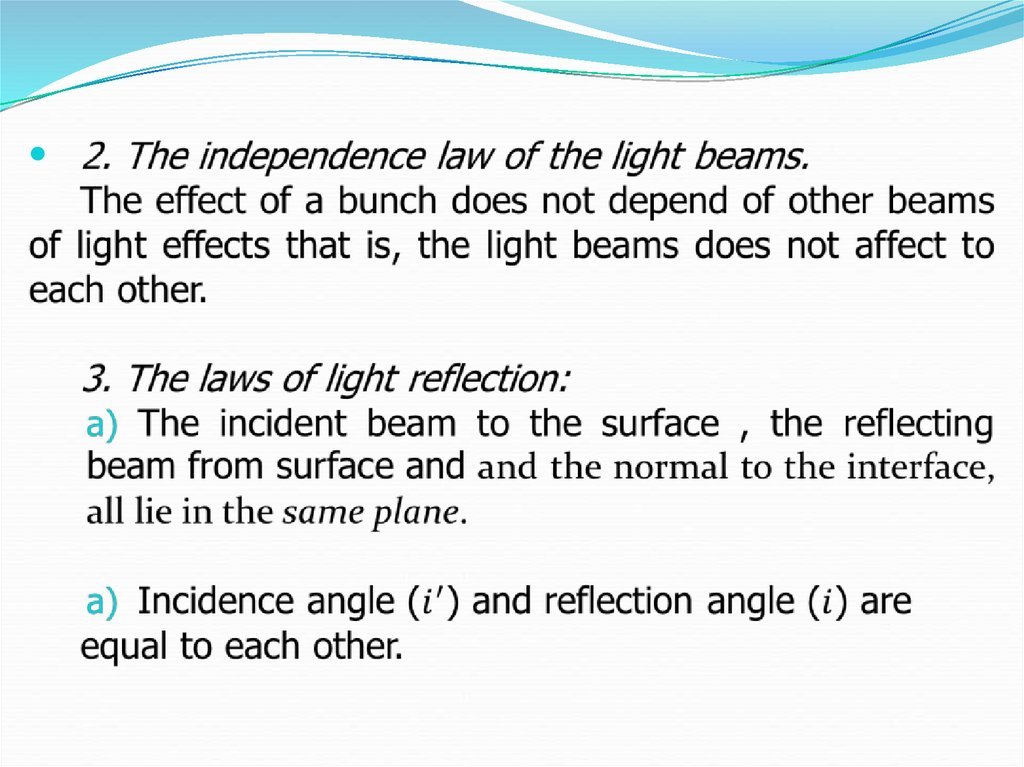# Fundamentals of ray optics. The laws of ray optics

## 2. Fundamentals of ray optics The laws of ray optics

Geometrical
optics,
or
ray
optics,
describes light propagation in terms of rays. The ray in
geometric optics is an abstraction, or instrument, useful in
approximating the paths along which light propagates in
certain classes of circumstances.
The assigned in practice the content of the laws of
beam optics:
1. The straight line of light propagation law.
The light beams propagate along a straight line in a
homogeneous medium. This law can be used only in cases
not accounted for only diffraction phenomena.

## 5. Refraction and reflection of light on a flat surface

1)The reflection of light on a flat mirror
The reflection of light from a plane mirror can be
summarised by the following laws:
1. The angle of incidence (i) is equal to the angle of
reflection (r)
2. The incident ray, reflected ray and the normal to the
surface at the point of incidence all lie in the same plane.

## 6.

Remember that with a glass mirror the reflecting
surface (usually a thin layer of aluminum or silver) is
placed on the back of the mirror and then covered
with a protective layer. This means that although the
main reflected image comes form this surface light will
also reflect from the front surface of the glass. This
will give a secondary image which is much weaker that
the main image. This is usually invisible in every day
life but would create severe problems in astronomy.
For this reason all astronomical mirrors have their
reflecting surface formed on the front of the glass.

## 8. The phenomenon of total internal reflection

Total internal reflection is a phenomenon which
occurs when a propagating wave strikes a medium
boundary at an angle larger than a particular critical
angle with respect to the normal to the surface. If
the refractive index is lower on the other side of the
boundary and the incident angle is greater than the
critical angle, the wave cannot pass through and is
entirely reflected. The critical angle is the angle of
incidence above which the total internal reflection occurs.

## 9.

When a wave reaches a boundary between different
materials with different refractive indices, the wave will in
general be partially refracted at the boundary surface, and
partially reflected. However, if the angle of incidence is
greater (i.e. the direction of propagation is closer to being
parallel to the boundary) than the critical angle – the
angle of incidence at which light is refracted such that it
travels along the boundary – then the wave will not cross
the boundary, but will instead be totally reflected back
internally. This can only occur when the wave in a
medium with a higher refractive index (n1) reaches a
boundary with a medium of lower refractive index (n2).
For example, it will occur with light reaching air from
glass, but not when reaching glass from air.

## 10.

Total internal reflection of light can be demonstrated
using a semi-circular block of glass or plastic. A "ray box"
shines a narrow beam of light (a "ray") onto the glass
medium. The semi-circular shape ensures that a ray
pointing towards the centre of the flat face will hit the
curved surface at a right angle; this will prevent refraction
at the air/glass boundary of the curved surface. At the
glass/air boundary of the flat surface, what happens will
depend on the angle.

## 11.

Where θc is the critical angle measurement which is
caused by the sun or a light source(measured
normal to the surface):
If θ < θc, the ray will split. Some of the ray will
reflect off the boundary, and some will refract as it
passes through. This is not total internal reflection.
If θ > θc, the entire ray reflects from the boundary.
None passes through. This is called total internal
reflection.
This physical property makes optical fibers useful
and prismatic binoculars possible. It is also what gives
diamonds their distinctive sparkle, as diamond has an
unusually high refractive index.